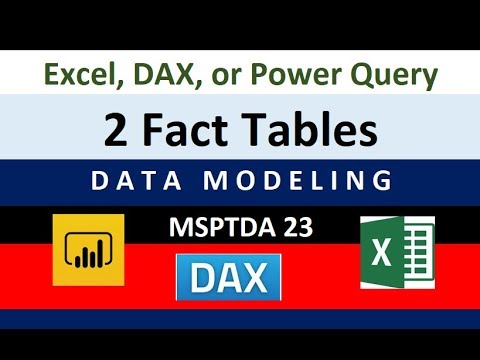Select Page

All 9 files for video in this zipped folder: https://people.highline.edu/mgirvin/AllClasses/348/MSPTDA/Content/DataModeling/MSPTDA-23-DownloadAllFiles.zip
Download file individually at class web site: https://people.highline.edu/mgirvin/AllClasses/348/MSPTDA/Content/DataModeling/DataModeling.htm
pfd notes for Video #23: https://people.highline.edu/mgirvin/AllClasses/348/MSPTDA/Content/DataModeling/023-MSPTDA-TwoFactTables.pdf

In this video learn about how to convert 2 Fact Tables into one with DAX, Worksheet Formulas or Power Query
Comprehensive Microsoft Power Tools for Data Analysis Class, BI 348, taught by Mike Girvin, Excel MVP and Highline College Professor.
Topics:
1. (00:16) Introduction

2. (02:35) Excel Worksheet Formula Solution
3. (05:01) How do we allocate Discount from Invoice Grain to Invoice Line Grain?
4. (05:45) Worksheet Formula for Total Invoice Sales at Invoice Grain using SUMPRODUCT function
5. (09:23) Worksheet Formula for % Sales Discount at Invoice Grain using division
6. (10:00) Worksheet Formula for Line Discount at Invoice Line Grain using VLOOKUP and multiplication
7. (12:12) How do we allocate Shipping from Invoice Grain to Invoice Line Grain?
8. (13:27) Worksheet Formula for Invoice Line Shipping Weight at Invoice Line Grain using VLOOKUP and multiplication
9. (14:30) Worksheet Formula for Invoice Weight at Invoice Grain using SUMIFS
10. (15:04) Worksheet Formula for Line Shipping at Invoice Line Grain using VLOOKUP and multiplication
11. (16:50) Create Excel Reports at Product Grain.
12. (17:03) Standard PivotTable Report
13. (17:53) Worksheet Formula Report

14. (21:26) DAX Formula Solution in Power Pivot
15. (22:30) Look at Data Model and preview of DAX Formulas and functions SUMX, RELATED and RELATEDTABLE
16. (24:30) Bring Excel Tables into Data Model
17. (25:15) Create Relationships between tables
18. (26:23) How to Allocate Invoice Grain Numbers to Invoice Line Grain Numbers
19. (26:56) DAX Formula for Total Invoice Sales at Invoice Grain using SUMX and RELATEDTABLE functions
20. (29:40) DAX Formula for % Sales Discount at Invoice Grain using DIVIDE function
21. (30:50) DAX Formula for Line Discount at Invoice Line Grain using RELATED function and multiplication
22. (31:57) DAX Measure for Total Discount
23. (32:39) Data Model PivotTable Report for Product Discount
24. (33:08) How do we allocate Shipping from Invoice Grain to Invoice Line Grain?+
25. (34:22) DAX Formula for Invoice Weight at Invoice Grain using SUMX, RELATEDTABLE and RELATED.
26. (35:56) Visuals to understand how DAX Formula with SUMX, REALTEDTABLE and RELATED are working to traverse multiple relationships in one formula. This helps illustrates the Power of DAX for Business Calculations.
27. (36:52) DAX Formula for Line Shipping at Invoice Line Grain using RELATED and multiplication and division. Three RELATED function in one formula
28. (39:03) DAX Measure for Total Shipping
29. (39:25) Final Data Model PivotTable

30. (40:22) Power Query Solution in Power BI Desktop
31. (41:09) Why Two Fact Tables will not work with all Dimension Tables for Reporting.
32. (43:05) Summary and visuals of steps we need to perform
33. (44:12) Create blank Power BI Desktop file
34. (44:40) Import Two Fact Table Data Model from Power Pivot
35. (45:40) Power Query Formula to calculate Sales at Invoice Line Grain using Table.AddColumn function
36. (46:53) Power Query Merge to lookup Product Weight at Invoice Line Grain
37. (47:26) Power Query Formula to calculate Product Shipping Weight at Invoice Line Grain using Table.AddColumn function
38. (47:42) Power Query Group By feature to aggregate Invoice Sales, Invoice Shipping Weight and all rows in Invoice Line Grain Table for each Invoice Number.
39. (49:52) Power Query Merge to pull Invoice Grain Shipping & Discount numbers, as well as to pull the Invoice Level Dimensions of Date and Sales Rep ID into the current step in the query (later after expanding it will be the Invoice Line Grain).
40. (50:52) Power Query Formula for % Sales Discount at Invoice Grain using Table.AddColumn function
41. (52:11) Expand to get back to Invoice Line Grain
42. (52:31) Note about Unit Price and how it is stored as a Fact because it changes so often.
43. (54:16) Power Query Formula for Line Discount at Invoice Line Grain using Table.AddColumn function and Number.Round
44. (54:49) Power Query Formula for Line Shipping at Invoice Line Grain using Custom Column using Table.AddColumn function and Number.Round
45. (57:23) Remove all column we do not need in final Fact Table
46. (58:08) Load Tables to Data Model, except Invoice Level Table.
47. (59:15) Create DAX Measures for Shipping, Discounts and Sales
48. (01:00:10) Create % DAX Measures for Shipping and Discount as a percent of sales. Use the DIVIDE DAX Function.
49. (01:01:15) Hide Columns from Report View
50. (01:01:33) Look at Final Data Model
51. (01:01:47) Create Visualization in Power BI Desktop
52. (01:04:40) SummaryRead More